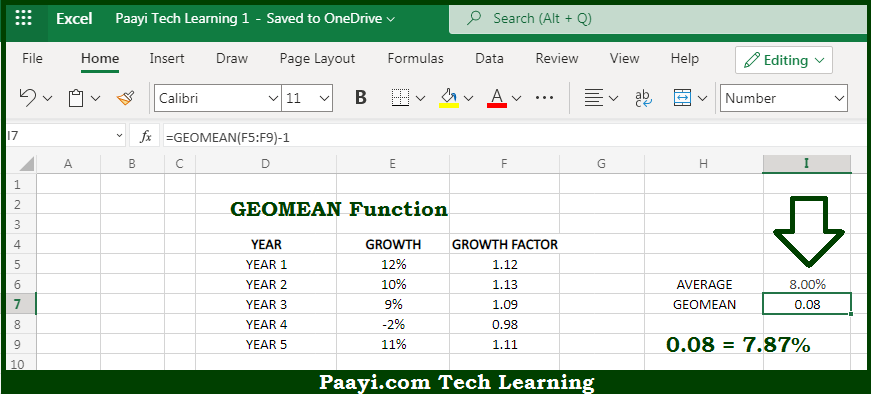# Learn How to Use Microsoft Excel GEOMEAN Function

Written by | 0 Comments | 681 Views

In this article, you will learn how to use the Microsoft Excel GEOMEAN function and its prime function in Microsoft Excel. You will also get to know the Microsoft Excel GEOMEAN function return value and syntax with the help of some examples.

Microsoft Excel GEOMEAN Function

The main purpose of the Microsoft Excel GEOMEAN function is used to calculate the geometric mean. That implies, with the help of the GEOMEAN function you can able to return the geometric mean for a set of numeric values. It should be noted that the Geometric mean can be used to calculate the average rate of return with variable rates. So, with the help of the GEOMEAN function, you can able to calculate the geometric mean.

Return Value of GEOMEAN Function

The return value will be the calculated mean.

Syntax of GEOMEAN Function

=GEOMEAN(number1, [number2], ...)

Where the arguments:

• number1: This is the first value or reference.
• number2: This is the second value or reference (optional).

## How to Use Microsoft Excel GEOMEAN Function?So we know that with Microsoft Excel GEOMEAN function you can able to calculate the geometric mean. That implies, with the help of the GEOMEAN function you can able to return the geometric mean for a set of numeric values. It should be noted that the Geometric mean can be used to calculate the average rate of return with variable rates. So, with the help of the GEOMEAN function, you can able to calculate the geometric mean.